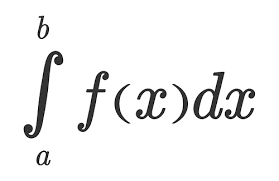# R as learning tool: solving integrals

May 5, 2018
By

Want to share your content on R-bloggers? click here if you have a blog, or here if you don't.Integrals are so easy only math teachers could make them difficult.When I was in high school I really disliked math and, with hindsight, I would say it was just because of the the prehistoric teaching tools (when I saw this video I thought I’m not alone). I strongly believe that interaction CAUSES learning (I’m using “causes” here on purpose being quite aware of the difference between correlation and causation), practice should come before theory and imagination is not a skill you, as a teacher, could assume in your students. Here follows a short and simple practical explanation of integrals. The only math-thing I will write here is the following: f(x) = x + 7. From now on everything will be coded in R. So, first of all, what is a function? Instead of using the complex math philosophy let’s just look at it with a programming eye: it is a tool that takes something in input and returns something else as output. For example, if we use the previous tool with 2 as an input we get a 9. Easy peasy. Let’s look at the code:

```# here we create the tool (called "f")
# it just takes some inputs and add it to 7
f <- function(x){x+7}

# if we apply it to 2 it returns a 9
f(2)
9

```

Then the second question comes by itself. What is an integral? Even simpler, it is just the sum of this tool applied to many inputs in a range. Quite complicated, let’s make it simpler with code:

```# first we create the range of inputs
# basically x values go from 4 to 6
# with a very very small step (0.01)
# seq stands for sequence(start, end, step)
```

```x <- seq(4, 6, 0.01)
x
4.00 4.01 4.02 4.03 4.04 4.05 4.06 4.07...

x
4

x
4.01
```

As you see, x has many values and each of them is indexed so it’s easy to find, e.g. the first element is 4 (x). Now that we have many x values (201) within the interval from 4 to 6, we compute the integral.

```# since we said that the integral is
# just a sum, let's call it IntSum and
# set it to the start value of 0
# in this way it will work as an accumulator
```
```IntSum = 0
```

Differently from the theory in which the calculation of the integral produces a new non-sense formula (just kidding, but this seems to be what math teachers are supposed to explain), the integral does produce an output, i.e. a number. We find this number by summing the output of each input value we get from the tool (e.g. 4+7, 4.01+7, 4.02+7, etc) multiplied by the step between one value and the following (e.g. 4.01-4, 4.02-4.01, 4.03-4.02, etc). Let’s clarify this, look down here:

```# for each value of x
for(i in 2:201){

# we do a very simple thing:
# we cumulate with a sum
# the output value of the function f
# multiplied by each steps difference

IntSum = IntSum + f(x[i])*(x[i]-x[i-1])

# So for example,
# with the first and second x values the numbers will be:
#0.1101 = 0 + (4.01 + 7)*(4.01 - 4)

# with the second and third:
#0.2203 = 0.1101 + (4.02 + 7)*(4.02 - 4.01)

# with the third and fourth:
#0.3306 = 0.2203 + (4.03 + 7)*(4.03 - 4.02)

# and so on... with the sum (integral) growing and growing
# up until the last value
}

IntSum
24.01
```

Done! We have the integral but let’s have a look to the visualization of this because it can be represented and made crystal clear. Let’s add a short line of code to serve the purpose of saving the single number added to the sum each time. The reason why we decide to call it “bin” instead of, for example, “many_sum” will be clear in a moment.

```# we need to store 201 calculation and we
# simply do what we did for IntSum but 201 times
bin = rep(0, 201)
bin
0 0 0 0 0 0 0 0 0 0 0 0 ...
```

Basically, we created a sort of memory to host each of the calculation as you see down here:

```for (i in 2:201){

# the sum as earlier
IntSum = IntSum + f(x[i])*(x[i]-x[i-1])

# overwrite each zero with each number
bin[i] = f(x[i])*(x[i]-x[i-1])
}

IntSum
24.01

bin
0.0000 0.1101 0.1102 0.1103 0.1104 0.1105 ..

sum(bin)
24.01
```

Now if you look at the plot below you get the whole story: each bin is a tiny bar with a very small area and is the smallest part of the integral (i.e. the sum of all the bins).

```# plotting them all
barplot(bin, names.arg=x)
```This tells you a lot about the purpose of integral and the possibility of calculating areas of curvy surfaces. To have an idea of this just change the function f with, let’s say, sin(x) or log(x). What is happening? And what if you increase/decrease the number of bins? Have fun replicating the code changing some numbers and functions. Integrals should be clearer in the end. That’s all folks! #R #rstats #maRche #Rbloggers This post is also shared in www.r-bloggers.com

R-bloggers.com offers daily e-mail updates about R news and tutorials about learning R and many other topics. Click here if you're looking to post or find an R/data-science job.
Want to share your content on R-bloggers? click here if you have a blog, or here if you don't.